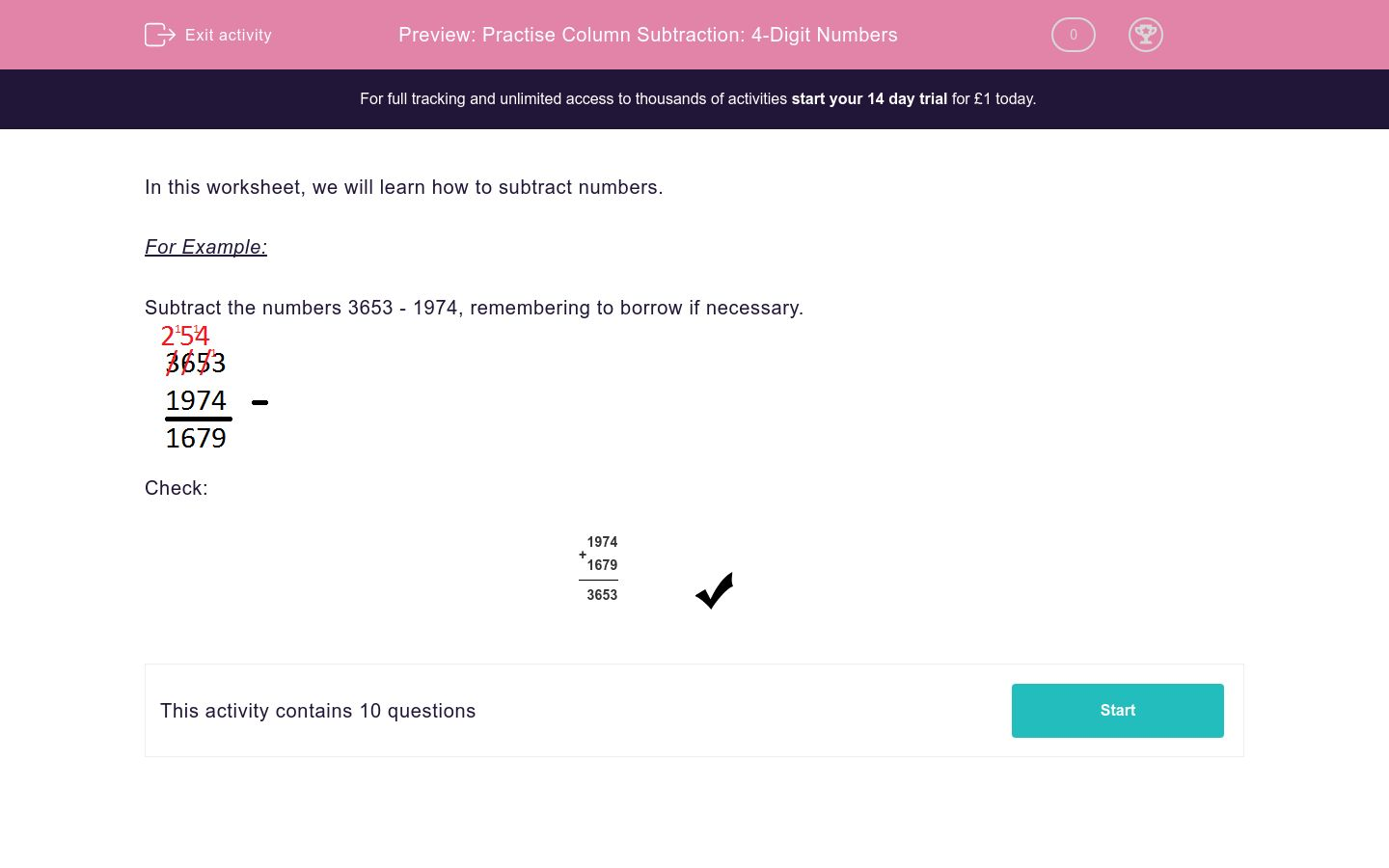# Practise Column Subtraction: 4-Digit Numbers

In this worksheet, students subtract four-digit numbers using columns with borrowing if necessary.Key stage:  KS 2

Curriculum topic:   Maths and Numerical Reasoning

Curriculum subtopic:   Mixed Problems

Difficulty level:### QUESTION 1 of 10

In this worksheet, we will learn how to subtract numbers.

For Example:

Subtract the numbers 3653 - 1974, remembering to borrow if necessary.Check:

 + 1 9 7 4 1 6 7 93 6 5 3

Subtract the numbers.

9878 – 2891  =  ___________

Subtract the numbers.

9603 – 1182  =  ___________

Subtract the numbers.

8724 – 2786  =  ___________

Subtract the numbers.

8915 – 6314  =  ___________

Subtract the numbers.

7517 – 3597 = ___________

Subtract the numbers.

8461 – 1075 = ___________

Subtract the numbers.

6113 – 4392 = ___________

Subtract the numbers.

9168 – 6068 = ___________

Subtract the numbers.

7790 – 1799 = ___________

Subtract the numbers.

5260 – 3917 = ___________

• Question 1

Subtract the numbers.

9878 – 2891  =  ___________

6987
• Question 2

Subtract the numbers.

9603 – 1182  =  ___________

8421
• Question 3

Subtract the numbers.

8724 – 2786  =  ___________

5938
• Question 4

Subtract the numbers.

8915 – 6314  =  ___________

2601
• Question 5

Subtract the numbers.

7517 – 3597 = ___________

3920
• Question 6

Subtract the numbers.

8461 – 1075 = ___________

7386
• Question 7

Subtract the numbers.

6113 – 4392 = ___________

1721
• Question 8

Subtract the numbers.

9168 – 6068 = ___________

3100
• Question 9

Subtract the numbers.

7790 – 1799 = ___________

5991
• Question 10

Subtract the numbers.

5260 – 3917 = ___________

1343
---- OR ----

Sign up for a £1 trial so you can track and measure your child's progress on this activity.

### What is EdPlace?

We're your National Curriculum aligned online education content provider helping each child succeed in English, maths and science from year 1 to GCSE. With an EdPlace account you’ll be able to track and measure progress, helping each child achieve their best. We build confidence and attainment by personalising each child’s learning at a level that suits them.

Get started